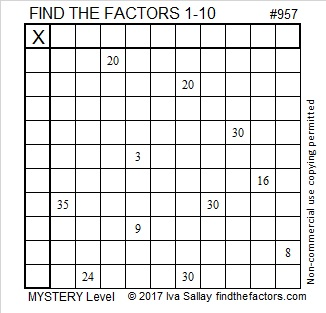# 957 Mystery Pentagon Puzzle

Pentagons have been on my mind this week so here is another puzzle with a pentagon in it. This time the pentagon is small. How difficult is this Mystery Level puzzle?  That depends on if you recognize one very important piece of logic needed to solve it. If you see that logic, it’s not too bad. If you don’t, it might do you in.Print the puzzles or type the solution in this excel file: 10-factors-951-958

957 is the hypotenuse of a Pythagorean triple:
660-693-957 which is (20-21-29) times 33

957 is repdigit TT in BASE 32 (T is 29 base 10)
because 29(32) + 29(1) = 29(32 + 1) = 29(33) = 957
957 is also T0 in BASE 33 because 29(33) + 0(1) = 957

• 957 is a composite number.
• Prime factorization: 957 = 3 × 11 × 29
• The exponents in the prime factorization are 1, 1, and 1. Adding one to each and multiplying we get (1 + 1)(1 + 1)(1 + 1) = 2 × 2 × 2 = 8. Therefore 957 has exactly 8 factors.
• Factors of 957: 1, 3, 11, 29, 33, 87, 319, 957
• Factor pairs: 957 = 1 × 957, 3 × 319, 11 × 87, or 29 × 33
• 957 has no square factors that allow its square root to be simplified. √957 ≈ 30.9354This site uses Akismet to reduce spam. Learn how your comment data is processed.